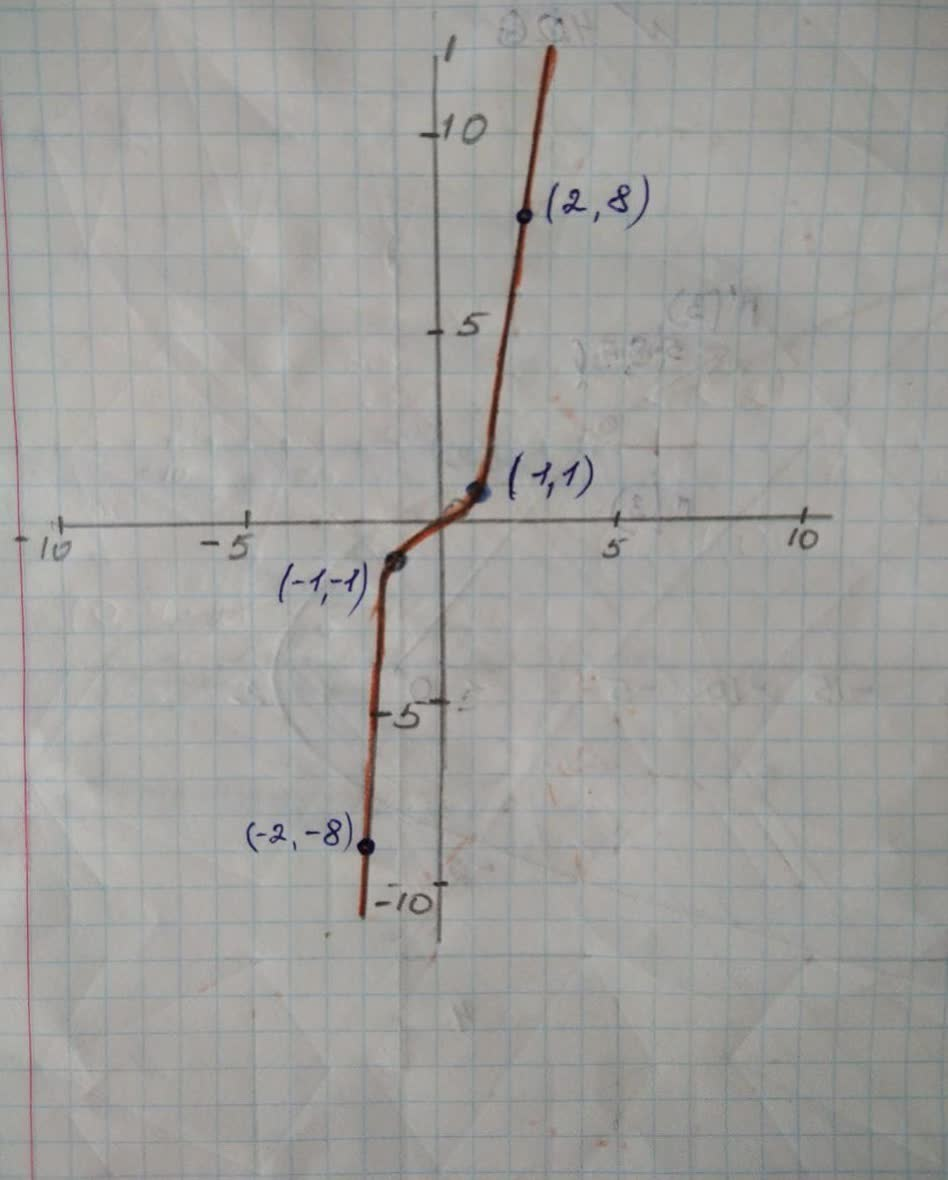# Let C be the curve described by the parametric equationsprelimaf1 2021-12-04 Answered
Let C be the curve described by the parametric equations $x\left(t\right)=t,y\left(t\right)={t}^{3}$.
a) sketch a graph of C.
b) find the vector-valued function f(t) associated with this parameterization of the curve C.
c) find the velocity vector and the speed of f(t) at t=1.
You can still ask an expert for help

• Questions are typically answered in as fast as 30 minutes

Solve your problem for the price of one coffee

• Math expert for every subject
• Pay only if we can solve itkayleeveez7

Step 1
Given equation is
$x\left(t\right)=t,y\left(t\right)={t}^{3}$
Step2
To sketch the curve let convert it into Cartesian form

$⇒y={x}^{3}$
Step3
Graph of curve Cis as shownStep4
b)
vector-valued function f(t) associated with this parameterization of the curve C.
$⇒f\left(t\right)={t}^{3}$
Step5
c) the velocity vector and the speed of f(t) at t=1.
$⇒{f}^{\prime }\left(t\right)=3{t}^{2}$

$⇒{f}^{\prime }\left(t\right)=3{t}^{2}$
$⇒{f}^{\prime }\left(t\right)=3×{1}^{2}$
$⇒{f}^{\prime }\left(t\right)=3$